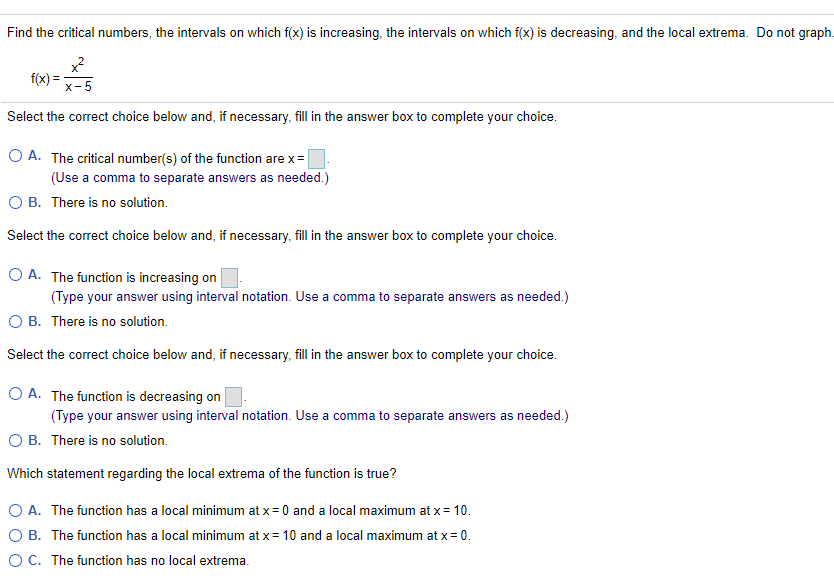### Create an Account

Already have account?

### Forgot Your Password ?

Home / Questions / Find the critical numbers, the intervals on which f(x) is increasing, the intervals on whi...

# Find the critical numbers, the intervals on which f(x) is increasing, the intervals on which f(x) is decreasing, and the local extrema. Do not graph f(x)= x? X-5 hoe Select the correct choice below an

Find the critical numbers, the intervals on which f(x) is increasing, the intervals on which f(x) is decreasing, and the local extrema. Do not graph f(x)= x? X-5 hoe Select the correct choice below and, if necessary, fill in the answer box to complete your choice. O A. The critical number(s) of the function are x = (Use a comma to separate answers as needed.) OB. There is no solution. Select the correct choice below and, if necessary, fill in the answer box to complete your choice. O A. The function is increasing on (Type your answer using interval notation. Use a comma to separate answers as needed.) OB. There is no solution. Select the correct choice below and, if necessary, fill in the answer box to complete your choice. O A. The function is decreasing on (Type your answer using interval notation. Use a comma to separate answers as needed.) O B. There is no solution. Which statement regarding the local extrema of the function is true? O A. The function has a local minimum at x = 0 and a local maximum at x = 10. OB. The function has a local minimum at x= 10 and a local maximum atx=0. OC. The function has no local extremaApr 14 2021 View more View Less

#### Answer (Solved)Subscribe To Get Solution